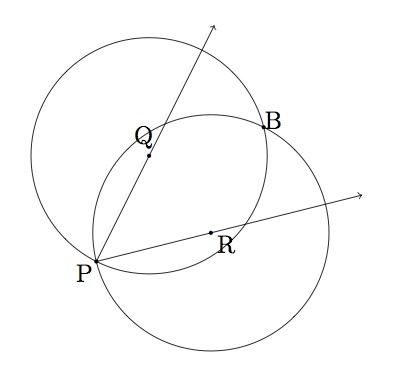# Bisecting an angle

Alignments to Content Standards: G-CO.D.12

Suppose $A$ is an angle with vertex $P$, as pictured below:1. Draw a circle with center $P$ and with radius $r \gt 0$. Explain why the circle meets each ray of angle $A$ in a single point. Label these points $Q$ and $R$ respectively.
2. Draw circles with centers $Q$ and $R$ respectively and radius $r$. These circles meet at $P$ and a second point to be labelled $B$. Show that ray $\overrightarrow{PB}$ bisects angle $P$.

## IM Commentary

This task provides the most famous construction to bisect a given angle. It applies when the angle is not $180$ degrees. Since the rays forming a $180$ degree angle are collinear, this case can be handled separately by constructing the perpendicular to a line through a given point: note that the construction in the collinear case is actually the same as the one employed here except that the two circles in step (b) need to have radii larger than $r$ so that they meet in two points.

For part (b) of the problem, it is not necessary to use $r$ for the radii of the circles with centers $Q$ and $R$. The only requirement is that the radii be chosen so that these two circles meet in two points. In practice, this means that the radius must be greater than half of $|QR|$. When dealing with apparent intersections of lines and circles, the teacher will likely wish to be very liberal in terms of what type of argument is considered acceptable. For example, in part (a), the definition of a ray through the vertex $P$ can be used to explain why a given circle with vertex $P$ and radius $r$ meets each ray in exactly one point. Alternatively, a picture could also be viewed as acceptable. In part (b), the circles of radius $r$ with centers $Q$ and $R$ definitely meet at $P$ (by construction) but finding the second point $B$ would require a sophisticated argument: for example, the equations of the two circles could be invoked and a long algebraic explanation could be given. In the context of this problem, however, using the picture which the students will draw with straightedge and compass is appropriate as an explanation.

We note that this construction comes historically from the compass-and-straightedge constructions, but are equally applicable in a setting where students use dynamic geometry software to explore geometry. This construction in particular could be used to develop students' geometric problem-solving skills, as they attempt to bisect an angle in a geometry program with only the most basic of tools available to them. As such, the task, which provides one solution to said construction problem, is principally intended for instruction or exploration purposes, although as a classic and elegant construction, all students should know this procedure for bisecting an angle.

This task includes an experimental GeoGebra worksheet, with the intent that instructors might use it to more interactively demonstrate the relevant content material. The file should be considered a draft version, and feedback on it in the comment section is highly encouraged, both in terms of suggestions for improvement and for ideas on using it effectively. The file can be run via the free online application GeoGebra, or run locally if GeoGebra has been installed on a computer.

The first two steps of this applet allow the user to change a couple things by dragging blue points. After these two steps, it is all a walk through.

## Attached Resources

• 1083 GeoGebra File
• ## Solution

1. For a positive number $r$ each ray through $P$ contains exactly one point of distance $r$ from $P$. So a circle of radius $r$ centered at $P$ will have one point of intersection with each ray, labelled $Q$ and $R$:2. Below is a picture of rays $\overrightarrow{PQ}, \overrightarrow{PR}$ and the circles of radius $r$ with centers $R$ and $Q$. Note that both of these circles contain $P$ because we know, from part (a), that both $Q$ and $R$ have distance $r$ from $P$. The second point of intersection is labelled $B$.We will use SSS to show that triangle $PRB$ is congruent to triangle $PQB$. This then shows that angle $RPB$ is congruent to angle $QPB$ and so the bisection of angle $A$ will be complete. We know that $|QP| = |RP| = r$ since these segments are both radii of a circle of radius $r$ and center $P$. We know that $|QB| = |RB| = r$ because these are both radii of a circles with radius $r$ and centers $Q$ and $R$ respectively. Finally, we have $|PB| = |PB|$ and so triangle $PRB$ is congruent to triangle $PQB$ as desired.